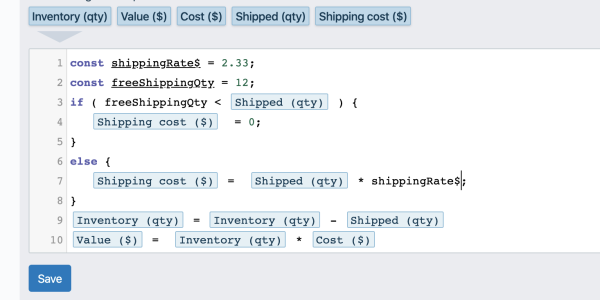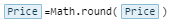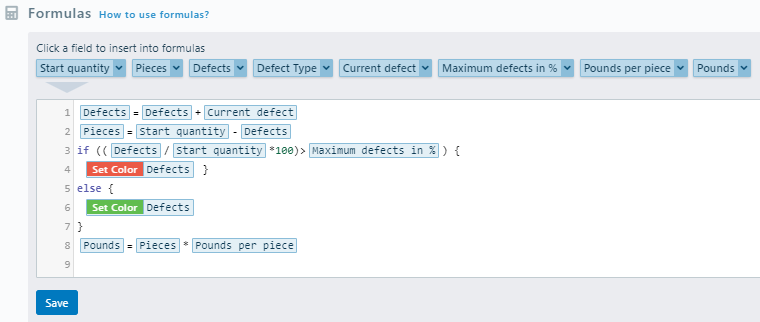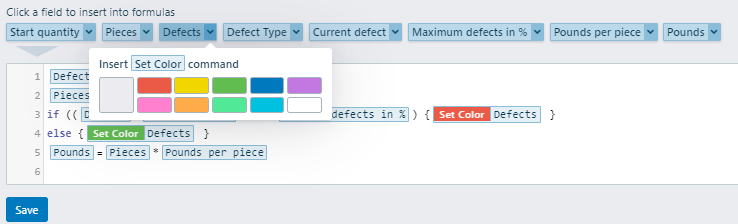# Advanced Formulas (It's actually Javascript)

You can use any and all tools that Javascript offers.

One of many, many resources on the subject of the Javascript.
https://www.w3schools.com/js/js_syntax.asp

Here is an example of using variables and conditions in the SmartFields formulas.You can use any and all Math functions https://www.w3schools.com/js/js_math.asp

For example to remove all decimals for a field just do this in your formulas:

You can use

Math.round() - to round mathematically: Math.round(3.33) -> 3 , Math.round(3.53) -> 4
Math.floor() - to round down: Math.round(3.33) -> 3
Math.ceil() - to round up: Math.ceil(3.33) -> 4Please pay attention that you should type exactly as in example Math.Round will not work !

#### Conditional coloring

Let's imagine that you would like to compare the value of the field with the reference value and if it exceeds the color of the field should be changed as an alertIn this example, we accumulating defects that occurred during production.

Then we check if the number of defects is more than Maximum allowed and if it is Set color to Red. If not let it be green.

To insert this command press on the arrow on the right side of the field name in Formulas section and choose a color.